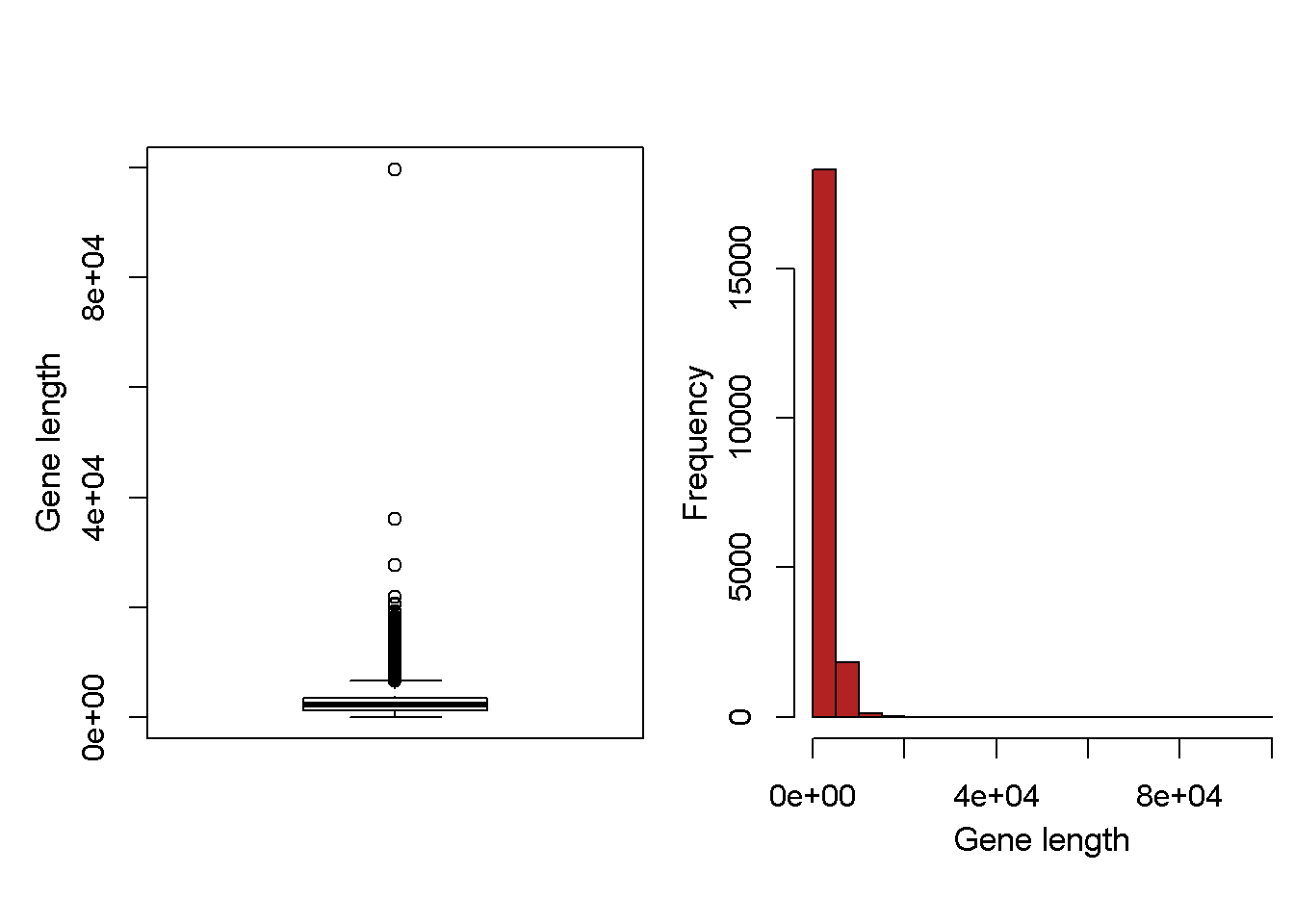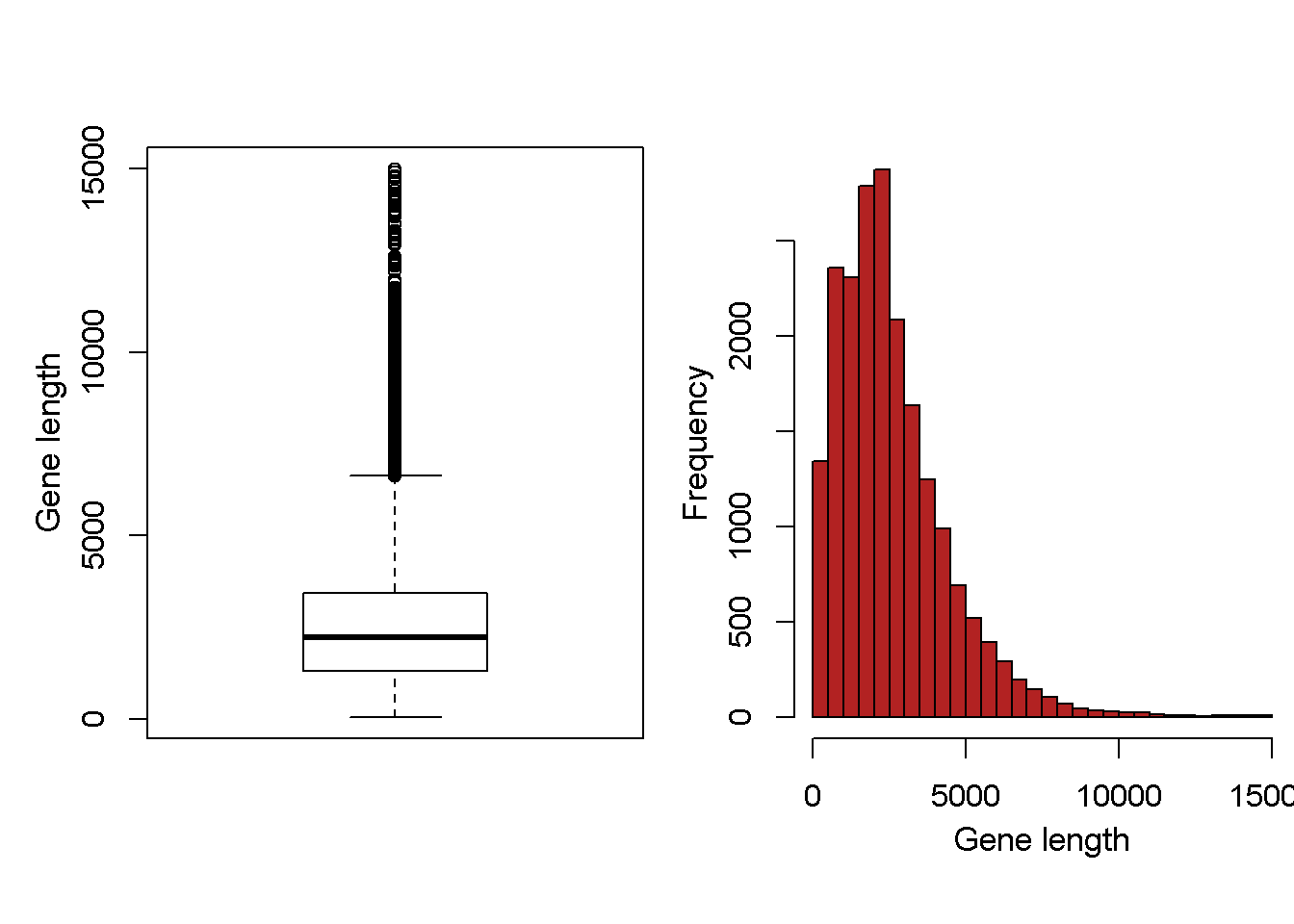Doing Math in R

• R can do everything a scientific calculator or spreadsheet can do. This includes basic functions like +, - , /, sqrt, etc, and basic functions like mean(), median(), sd() for the standard deviation and var() for the variance.

• In general in this course we will focus on having do R most of the calculations for our stats. However, its important to understand some of the underlying math.

• Today, to practice doing math in R and to learn about basic statsitcal functions, we’ll do calcualtions of the mean, variance, “sum of squares”, and standard devition “by hand” in R. We’ll then compare them to the output R produces.

• The data we’ll use for this is discussed in Chapter 4 of Whitlock & Schluter. The author’s accessed information on the human genome about the length of every gene that has been identified. This results in a list of 20290 genes and their length in DNA bases pairs (A, T, C and Gs).

• These genes vary in length from 60 bp to 99631

• mean = 2622.0274027 bp

• median = 2226.5 bp.

Distribution of genes in Human Genome

The distribution of genes looks like thisRevised Genome size distribution w/o outliers

There are some huge outliers so we’ll take the 26 largest genese out (as the authors do) and replot the data.

(NOTE: removal of outliers should only be done for a good reason and should be well-documented so that anyone that looks at your work or uses the data in the future knows what you did)Doing math with human genes

I took a random smaple of these genes for use to do math with.

Note: This was a truly random sample - every one of the >20000 genes had an equal chance of being included.

I’ll store this random sample of genes in an R “object” called “dr.Ns.genes”. The “<-” is an R function called the “assignment” operator that says “take this list of numbers and store it in the place called dr.Ns.genes”

Random sample of 10 genes stored in R object “dr.Ns.genes”

dr.Ns.genes <- c(1042,1472,4339,1865,4706,2439,3369,2988,3286, 3773)

It Doesn’t matter what I call the object as long as the name of the object starts with a letter, has NO SPACES, and only has periods (.) or underscores (_)

For example, all of these names for an object work.

drNsgenes <- c(1042,1472,4339,1865,4706,2439,3369,2988,3286, 3773)

dr_Ns_genes <- c(1042,1472,4339,1865,4706,2439,3369,2988,3286, 3773)

DR_Ns_GENES <- c(1042,1472,4339,1865,4706,2439,3369,2988,3286, 3773)

Its good practice to use informative names for R objects so you can remember what they are.

The basic math stuff in R

The summed length of the 10 randomly selected gene lengths

sum(dr.Ns.genes)
##  29279

The mean of the 10 randomly selected gene lengths

mean(dr.Ns.genes)
##  2927.9

The varinace (var)

var(dr.Ns.genes)
##  1470944

The standard deviation (sd)

sd(dr.Ns.genes)
##  1212.825

Note that the standard deviation is just the square root of the of the variance

#SD using R's function
sd(dr.Ns.genes)
##  1212.825
#SD as the sqrt of the variance
sqrt(var(dr.Ns.genes))
##  1212.825

The min, max, etc.

min(dr.Ns.genes)
##  1042
max(dr.Ns.genes)
##  4706
sum(dr.Ns.genes)
##  29279

Doing math for stats by hand

I’m going to change the name of my R object, or rather make a new R object that has the same data as dr.Ns.genes.

Calculating the mean gene length by hand

The numerator

my.sum <- sum(my.genes)

The denomintor

my.N <- length(my.genes)

The mean

my.mean <- my.sum/my.N

Same thing to get the mean, just in 1 step

my.mean <- sum(my.genes)/length(my.genes)

We can check our answer like this using the “==” function which asks R “are these two objects EXACTLy the same?”

my.mean == mean(my.genes)
##  TRUE

We can do the same thing to check that the standard deviation is indeed the square root of the mean

sd(dr.Ns.genes) == sqrt(var(dr.Ns.genes))
##  TRUE

If the were not the same, R would say “FALSE”

• Because R is very very very very precise any rounding errors will result in R saying that two things are NOT the same. Sometimes some R functions do do some rounding so you have to check “FALSE” sometimes.

Calcualting variance & standard deviation by hand, step by step

Variance and standard deviation are a fundamental quantity in statistics.

We’ll step through each part ofthe calculation

“Deviations” between the mean and each observation

Calculate the difference between each observation and the mean of all observation that you calculated above.

Note that here, R is doing math on a set of 10 numbers in the object “my.genes” and a single number, the mean, in “my.mean”

Yi.deviations <- my.genes-my.mean

Start making a dataframe (df)

We can keep track of the math by making a spreadsheet-like object in R called a matrix or dataframe using the cbind() command. cbind() means “column bind”

my.df <- cbind(my.genes,      #original list of gene lengths
Yi.deviations) #

Look at the matrix. Note that some of the deviations are positive and some are negative.

my.df
##       my.genes Yi.deviations
##  [1,]     1042       -1885.9
##  [2,]     1472       -1455.9
##  [3,]     4339        1411.1
##  [4,]     1865       -1062.9
##  [5,]     4706        1778.1
##  [6,]     2439        -488.9
##  [7,]     3369         441.1
##  [8,]     2988          60.1
##  [9,]     3286         358.1
## [10,]     3773         845.1

Calculate the“Squared deviations” between the mean and each observation

Take your set of deviations and square them using “^2”

Here, we’ve done math on a whole list of numbers at the same time.

Yi.deviations.square <- Yi.deviations^2

<br.

Squaring is key b/c it makes deviations greater than the mean and less than the mean equivalent. That is, we go from “deviations” that are positive and negative to “squared deviations” that are all negative.

A the square deviations to the dataframe

my.df <- cbind(my.df, Yi.deviations.square)

my.df
##       my.genes Yi.deviations Yi.deviations.square
##  [1,]     1042       -1885.9           3556618.81
##  [2,]     1472       -1455.9           2119644.81
##  [3,]     4339        1411.1           1991203.21
##  [4,]     1865       -1062.9           1129756.41
##  [5,]     4706        1778.1           3161639.61
##  [6,]     2439        -488.9            239023.21
##  [7,]     3369         441.1            194569.21
##  [8,]     2988          60.1              3612.01
##  [9,]     3286         358.1            128235.61
## [10,]     3773         845.1            714194.01

Sum of squares between the mean and each deviation

we can now calcualte the “sum of square deviations” or the “sum of squares”. This is the numerator (the thing on top) in the variance and standard deviation equations.

my.sum.of.squares<- sum(Yi.deviations.square)

This is a rather big number and I’m glad we don’t have to calcualte it by hand : )

my.sum.of.squares
##  13238497

And now…the variance

We can now use the sum of squares(SS) to calcuate the variance. This is is the SS divided by the sample size minus one. Recall that we calcualted the sample size above and put it in an object called “my.N”. We subtract n to get what is known as the “Degrees of freedom”. More on this later

#The sample size
my.N
##  10
#degrees of freedom
dfs <- my.N - 1

#The var
my.var <- my.sum.of.squares/dfs

#Could also do it more directly
my.var <- my.sum.of.squares/(my.N - 1)

And now…the standard deviation

The standard deviation is just the square root of the variance.

$\sigma = \sqrt{\frac{\sum\limits_{i=1}^{n} \left(x_{i} - \bar{x}\right)^{2}} {n-1}}$

my.sd <- sqrt(my.var)

We can check if our results are the same as R

#Variance
var(my.genes)
##  1470944
my.var
##  1470944
#stdev
sd(my.genes)
##  1212.825
my.sd
##  1212.825

We can check this also like this usig “==”

#Variance
var(my.genes) == my.var
##  TRUE
#stdev
sd(my.genes) == my.sd
##  TRUE

The standard error (SE)

The standard error is a fundamental quantity in stats. It is used as a measure of variation in sample data and is useful for making error bars for means. Is is the standard deviation divided by the square root of the sample size.

my.se <- my.sd/sqrt(my.N)